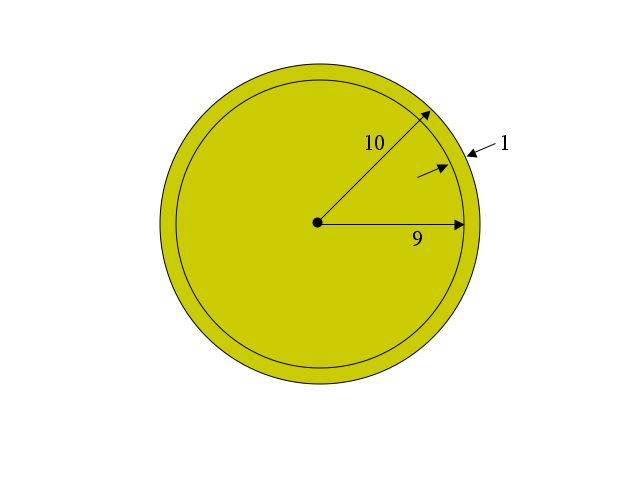Spheres in High Dimensions, Part 2

This continues the discussion from Part 1 on hyperspheres, spheres in high dimensional space (hyperspace). As a brief reminder of the problem statement, we are confronted with a 100-dimensional sphere of radius 10 made of solid gold and asked to choose between two pieces:

• the inner sphere of radius 9 of solid gold, or
• the outer shell of thickness 1 (inner radius 9, outer radius 10)

The two pieces between which we must choose are shown in the two-dimensional cross-section below, repeated from Part 1.While Part 1 answered the question of whether to choose the inner sphere of radius 9 or the outer shell of thickness 1, there are some interesting extensions.

First, while the approach taken in Part 1 is the one most students take and is therefore the most logical way to explain the solution, there is a simpler approach that can be found after some study. New results in mathematics often work this way. The person who discovers a new result often has to take a circuitous route to get there. But, once at that vantage point, he can look back and see a simpler approach. Naturally, when the result is published, only the simpler approach is presented, leaving us in even greater awe and wonder than is warranted.

I was tempted to skip the slightly more cumbersome derivation of Part 1 and make the following, simpler version the only basis of my explanation. But that would have given you a false impression of how most people, myself included, actually first solve the problem.

Returning for a moment to the 3 dimensional problem, the trick in the simpler derivation involves a minor rephrasing of the question. Instead of seeing which of the two pieces is larger, find if the inner sphere has more or less than half the total gold. Either way, you know which piece is larger.

So, instead of (as in Part 1) computing the ratio

V(shell ) / V(inner) = {[(4/3) π 103] - [(4/3) π 93]} / [(4/3) π 93]

and comparing it to 1 to see which of the two pieces is larger, consider the ratio

V(inner ) / V(total)

where V(total) is the total volume of both parts together. Since together, the two pieces form a solid sphere of radius 10,

V(total) = [(4/3) π 103]

and the new ratio

V(inner ) / V(total) = [(4/3) π 93] / [(4/3) π 103]

V(inner ) / V(total) = 93/103 = 0.93 = 0.729

Now it is follows directly that 72.9% of of the total one million dollars worth of gold, or \$729,000, is located in the inner sphere of radius 9, with the remaining \$271,000 being in the outer shell. Naturally we take the inner sphere in three dimensions.

Looking at the 93/103 in the last equation above, only three numbers appear: 9, 10 and 3. That was in 3-dimensions with radii of 9 and 10. So, extending to 100 dimensions (still with radii 9 and 10), it is reasonable to guess that the answer will be the same except that 100 will replace the 3:

V(inner ) / V(total) = 9100/10100

= 0.9100 = 0.00002656

which directly tells us that \$26.56 worth of gold is in the inner sphere, and the remaining \$999,973.44 is in the outer shell.

If you're still having fun with this problem, feel free to go on to Part 3 whenever you're ready.